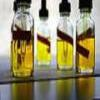#### You may also like### Mathematical Issues for Chemists

A brief outline of the mathematical issues faced by chemistry students.### Reaction Rates

Explore the possibilities for reaction rates versus concentrations with this non-linear differential equation### Catalyse That!

Can you work out how to produce the right amount of chemical in a temperature-dependent reaction?

# pH Temperature

##### Age 16 to 18Challenge Level

Water naturally dissociates into an equilibrium mixture of $H^+$ and $OH^-$ ions and $H_2O$ molecules
$$H_2O \rightleftharpoons^{K_W} H^++OH^-\,,$$
where the concentrations of $H^+$ and $OH^-$ ions, written as $[H^+]$ and $[OH^-]$ are related by the expression
$$K_W = [H^+][OH^-].$$
$K_W$ is called the dissociation constant, and depends on the temperature of the water.
The following table of data shows the dissociation constant for water at various temperatures and standard pressure.

 Water temperature $\quad K_W\times10^{14}\quad$ $0^\circ$ C 0.1 $10^\circ$ C 0.3 $18^\circ$ C 0.7 $25^\circ$ C 1.2 $30^\circ$ C 1.8 $50^\circ$ C 8.0 $60^\circ$ C 13 $70^\circ$ C 21 $80^\circ$ C 35 $90^\circ$ C 53 $100^\circ$ C 73

From this table, work out an estimate for the temperature at which water has a $pH$ of exactly 7, 6.8 and 7.2. Recall that the $pH$ is defined as $pH=-\log_{10}([H^+])$